Reach Us+44-1522-440391
Automatic Cycle Identification in Tidal Breathing Signals | OMICS International
Journal of Bioengineering & Biomedical Science

Like us on:

All submissions of the EM system will be redirected to Online Manuscript Submission System. Authors are requested to submit articles directly to Online Manuscript Submission System of respective journal.

# Automatic Cycle Identification in Tidal Breathing Signals

Yanwu Ding1*, Douglas F. Parham2, Zuojun Wang1 and Kanghee Lee1

1Department of Electrical Engineering and Computer Science, Wichita State University, 1845 N. Fairmount, Wichita, KS 67260, USA

2Department of Communication Sciences and Disorders, Wichita State University, 1845 N. Fairmount, Wichita, KS 67260, USA

*Corresponding Author:
Yanwu Ding
Department of Electrical Engineering and Computer Science
1845 N. Fairmount, Wichita, KS 67260, USA
E-mail: [email protected]

Received Date: January 19, 2012; Accepted Date: February 14, 2012; Published Date: February 17, 2012

Citation: Ding Y, Parham DF, Wang Z, Lee K (2012) Automatic Cycle Identification in Tidal Breathing Signals. J Bioeng Biomed Sci 2: 113. doi: 10.4172/2155-9538.1000113

Copyright: © 2012 Ding Y, et al. This is an open-access article distributed under the terms of the Creative Commons Attribution License, which permits unrestricted use, distribution, and reproduction in any medium, provided the original author and source are credited.

Visit for more related articles at Journal of Bioengineering & Biomedical Science

#### Abstract

In this paper, we introduce a novel cycle identification algorithm using MATLAB programming to automatically identify cycles in tidal breathing signals. The algorithm was designed in four steps using filtering, derivation, and other signal processing techniques. To verify the accuracy of the proposed algorithm, its results were compared with those of cycles identified manually by a human coder. Simulations results showed that despite the complexity of respiratory signals, the proposed algorithm could identify cycles more accurately than the human coder. This algorithm could serve as an important first step toward timely identification and coding for more complex respiratory signals, such as those underlying speech productions.

#### Keywords

Respiration; Respiratory signal; Inspiratory phase; Expiratory phase; Automatic cycle identification algorithm; Handcoded method; Cycle duration; Distorted cycle detection

#### Introduction

The analysis of periodic and quasiperiodic waveforms typically involves some form of automatic cycle detection [1-5]. There are powerful algorithms available to isolate specific frequencies of interest in a given waveform; many of these provide readily available measurements [6-8]. In the case of physiological behavior, rate measurements are often used as summary statistics of the waveforms representing that behavior (e.g. number of breaths per minute) . Unfortunately, cycle averaging techniques are often inadequate when it comes to describing complex behavior, particularly when that behavior changes over time [10,11]. In the case of respiratory behavior, at least two factors preclude the use of simple cycle averaging: (a) the detection of individual and physiologically informative variation in chest wall kinematics, and (b) the discrimination of interpretable and uninterpretable cycles. Both of these factors can be mitigated by the expertise of an experienced coder who can evaluate each cycle of a given waveform to determine which ones are acceptable, and then measure them accordingly. The downside to human visual coding and measurement is the time required to complete it. The twofold purpose of this inquiry is to design an algorithm that automatically detects tidal breath cycles across a variety of human subjects and to compare the algorithm’s performance to that achieved by an experienced human coder.

The proposed Automatic Cycle Identification Algorithm (ACIA) was used for tidal breath signals. The algorithm was designed in four steps using filtering, derivation, and other signal processing techniques via MATLAB programming [12,13]. To facilitate further analysis for tidal breath signals, the algorithm produced the exact start time for each cycle and isolated the distorted cycles due to artifacts. Simulations results have shown that, despite the inter- and intra-participant variability of the tidal breath signals, the proposed algorithm can identify tidal breath cycles correctly and accurately. It can also isolate those portions of the signals negatively impacted by motion artifact.

The paper is organized as follows. Section II details the respiratory signals used for this analysis. Section III describes the details of the steps in proposed algorithm. Section IV shows simulations to compare the results obtained by the proposed algorithm with hand-coded results obtained by the third author. Section V concludes the paper.

Notation: The uppercase letter M represents the total number of data samples in a tidal breath data file. The excursion voltage value at the m-th sample is denoted by Y(mτ), 0 ≤ m ≤ M-1, where τ is the sampling period in seconds (the sampling period in the analyzed data was 0.01seconds). The total number of cycles is denoted by NA, where subscript “A” is used to indicate the step and stage addressed in the proposed cycle identification algorithm. Typical values for “A” are I, II, or III. The start time for the n-th tidal breath cycle is denoted by TA[n]. The cycles are indexed consecutively; therefore, the start time for the n-th cycle is also the ending-time for the (n-1)-th cycle. Notation Ф[n] represents the maximum excursion voltage value in the n-th cycle. LA[n] and RA[n] denote, respectively, the minimum values in the inspiratory and expiratory phases in the n-th cycle (LA[n] and RA[n] are usually not identical). The letters L and R are used because the inspiratory phase is in the left portion of a cycle and the expiratory phase is in the right portion. LA[n] and RA[n] are also referred to as the left and right minimum excursion voltage in the n-th cycle. ΛA[n] and ΓA[n] denote, respectively, the inspiratory excursion difference and the expiratory excursion difference in the n-th cycle.

#### Overview of Tidal Breath Signals

The respiratory kinematic data used for algorithm development (Section III) and for evaluating the algorithm’s accuracy (Section IV) were taken from a separate and independent study by the third author exploring tidal breathing in twenty healthy women between 22 and 34 years of age. The participants’ respiratory behaviors were completely voluntarily (i.e., they were not paced or controlled by a metronome or other pattern-regulating device). The rationale for including only women in that study was to test hypotheses introduced in previous literature related to participants’ breath support during listening tasks. Each participant’s respiratory movements were measured using a respiratory inductive plethysmograph (Inductotracer, Ambulatory Monitoring, Inc., Ardsley, NY). The system produced two signals representing rib cage and abdomen excursions, the sum of which was calibrated to a known volume and adjusted using least-squares estimation.

Figure 1 shows two 12-second segments of tidal breath signals. Each tidal breath cycle is composed of an inspiratory phase and an expiratory phase, with the two phases being delineated by the maximum peak of each cycle. The inspiratory start and the expiratory end of each cycle are indicated by the vertical dashed lines. As demonstrated in the figure, although the tidal breath cycles are similar in their overall shape and quasi-periodic nature, the segments demonstrate some degree of inter- and intra-participant variability. Hand-coding the signal by an experienced coder is time-consuming and may be less precise than an algorithm-based identification system, depending on the resolution at which the signal is viewed and coded.

Figure 1: Examples of tidal breath signals. Start and end points of each cycle are marked by the vertical dashed lines. See Section II for more detail.

More examples of the variability of tidal breath cycles are provided in Figure 2. What is apparent is the difference in the ending segments of the expiratory phases among participants (indicated by the dotted boxes). The ripples within the signals are normal variations in chest wall movement, and a particular pattern can often be characteristic of an individual’s tidal breath signal. This is challenging for the algorithm design, as the ripples at the ending segments of the expiratory phases vary in their respective magnitudes and durations. The start times of the cycles are possibly located between any of those ripples or at the last ripple, as shown in the first graph in Figure 1 and in Figure 2. It is important that an algorithm-based coding system be able to adjust to and manage this variability across individual signals. As with most physiological signals, the signal representing chest wall movement is prone to distortion by motion artifact, which is caused primarily by gross-motor movement and/or shifts in posture that are transferred to the sensors. Figure 3 shows two examples of how motion artifact can negatively impact the interpretability of the tidal breath signal. The unwanted displacement or distortion of the signal makes it impossible to determine the end point of the previous cycle, which is equivalent to the starting point of the subsequent cycle. Motion artifact can also introduce short-term upward or downward trends in the signal (as can be seen in the lower graph of Figure 3). It is not difficult for a human coder to identify, isolate, and remove from analysis the presence of motion artifact in the tidal breath signal; an algorithm-based coding system must be able to do the same.

Figure 2: Examples of individual tidal breath cycles. Start and end points of each cycle are marked by the vertical dashed lines. Normally occurring variations in the end portion of the expiratory phase are identified by the dotted boxes. See Section II for more detail.

Figure 3: Examples of the impact of motion artifact on tidal breath signals. The signal displacement caused by gross-motor movement-and its impact on the subsequent cycle-is identified within the dashed boxes. See Section II for more detail.

#### Automatic Cycle Identification Algorithm

The proposed automatic cycle identification algorithm included four steps as shown in Figure 4. The first three steps focused on cycle identifications, including determining the total number of cycles and their exact start times. The fourth step detected and isolated the distorted cycles that cannot be used for the purpose of respiratory signal analysis. We first give an overview of the four steps with illustrating figures, followed by a detailed description of each step in the algorithm. A subset of the tidal breath signals described in Section II (six out of twenty participants) was used to develop the algorithm.

Overview of the algorithm

Step I. Identification of all possible cycles: The goal of Step I was to identify all possible cycles in a tidal breath data file by looking for possible start times via initial processing techniques, including decimation, derivation, and interpolation. The cycles were marked by vertical lines drawn at the start time for each cycle, as indicated in the first plot in Figure 5. As the cycles were numbered consecutively from the beginning of the data, the start time of a cycle was also the end time of the previous cycle. It was clear from Figure 5 that the initial processing techniques in Step I also identified a number of cycles between two normal or desired cycles. As these cycles did not represent any normal tidal breath cycles, they were referred as “undesired cycles” and needed to be removed. This task was carried out in Step II.

Figure 5: Corresponding cycle identification results of Automatic Cycle Identification Algorithm. Distorted cycles represent segments of the signal that could not be associated reliably with a physiological event. See also Figure 4.

Step II. Removal of undesired cycles: It was observed that the undesired cycles identified in Step I could generally be classified into two patterns based on their locations. As shown in the second plot in Figure 5, the patterns were represented by the dashed and dotted-dashed cycle identification lines, respectively. Two criteria were proposed to remove the undesired cycles using various characteristics of tidal breath signals such as local maxima, minima, and slope. After the undesired cycles were removed, the cycles were renumbered. The index of the last cycle was also the total number of desired cycles identified in this step.

Step III. Adjustment of start time for the cycles: By implementing Step II, all the desired cycles were identified successfully by their start times and marked by vertical lines. However, there was often a small offset between the correct start time and the time marked by the vertical line obtained in Step II. Therefore, Step III was designed to find the exact start time of each cycle and perform further adjustment on the corresponding identification line. Due to the complexity in tidal breath signals, a two-stage adjustment procedure was designed. In order to locate the correct start time, the time at which the next inspiratory phase started was identified and the local minimum in the end portion of the expiratory phase between two adjacent cycles was examined. The third plot in Figure 5 shows the results obtained in Step III.

Step IV. Distorted cycle detection: As mentioned in Section II, some cycles in the signals were distorted due to motion artifacts. Examples for those cycles were plotted by dashed lines in the last graph in Figure 5. The distorted cycles had undesired upwards or downwards displacement. Those cycles could not be used for the purpose of future analysis because it was impossible to identify the ending points of the previous cycles. Step IV was designed to detect, isolate and remove the distorted cycles.

Detailed description of the algorithm

Step I. Identification of all possible cycles: Although the respiratory signals were roughly quasiperiodic with each cycle resembling a sinusoidal-squared waveform, the time duration for each cycle was not a fixed constant. Furthermore, the excursion voltage values at the end of expiratory phases often fluctuated rapidly in small magnitudes. These observations motivated us to use derivation as an initial means to identify the start time for possible cycles. As demonstrated in Figure 6, the start time for the n-th cycle was identified by a vertical line located at time TI[n], where subscript “I” denoted Step I. In the proposed algorithm, the signal was decimated by a factor of 30. Interpolation was performed on the decimated respiratory signal to recover the original time duration.

Figure 6: Step I of Automatic Cycle Identification Algorithm. Desired cycle identification represents first step of algorithm.

The signals in the end portion of an expiratory phase and the next inspiratory phase usually did not possess any mathematically tractable waveforms. Furthermore, the signals were difficult to analyze if there was motion artifact in the end portion of the expiratory phase (see Figure 2). As a result, by taking the derivative of the respiratory signals, small bumps in the signal were identified as cycles by Step I as shown by dashed lines in Figure 6.

Step II. Removal of undesired cycles: To describe details in the algorithm, we first introduced the following measurements associated with Step A (depending on which step was being addressed, “A” took the value I, II, III, or IV). Figure 7 illustrates these measurements.

Figure 7: Cycle measurements made in Automatic Cycle Identification Algorithm. See Section III-B2 for details.

ФA[n] was the maximum excursion voltage in the n-th cycle in Step A: ФA[n] = max(Y(t), TA[n] ≤ t ≤ TA[n+1]), where TA[n] and TA[n+1] were, respectively, the start times of the n-th and (n+1)-th cycle obtained in Step A (here symbol “A” takes value of I, II, III, IV,…). The time at which the maximum voltage occurs was denoted by T A*[ n] , i.e. ΦA [ n] = Y (TA *[n]) .

LA[n] was the minimum inspiratory excursion voltage in the n-th cycle in Step A: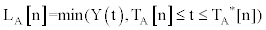. As the inspiratory phase was located at the left portion of a tidal cycle, it was also referred to as the “left minimum voltage” (indicated by “L”).

RA[n] was the minimum expiratory excursion voltage in the n-th cycle in Step A: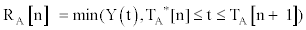. It was also referred to as the “right minimum voltage” (indicated by “R”).

ΛA[n] was the inspiratory excursion difference in the n-th cycle in Step A: ΛA[n] = ФA[n]-LA[n]. It was calculated by the difference between maximum excursion voltage ФA[n] and left minimum voltage LA[n].

ГA[n] was the expiratory excursion difference in the n-th cycle in Step A: ГA[n] = ФA[n]-RA[n]. It was calculated by the difference between maximum excursion voltage ФA[n] and right minimum voltage RA[n].

The identification lines for these undesired cycles were typically drawn either at the end portion of the expiratory phase between two desired adjacent cycles if there were bumps in the end portion of the expiratory phase or inside a cycle if there existed small dips in the inspiratory phase. The undesired cycles could be classified into two patterns by their locations. Pattern 1 classified the undesired cycles located in the end portion of the expiratory phase between two adjacent normal tidal breath cycles. Figure 8 shows examples of undesired cycles (marked by dashed lines) in Pattern 1. The voltage difference between the maximum and minimum voltages in these undesired cycles was much smaller than those in desired cycles. Pattern 1 often occurred when participants had longer expiratory phases and the expiratory voltages fluctuated more rapidly. As a result, more than one undesired cycle was often identified by Step I due to the multiple bumps. In Pattern 2, the undesired cycles were typically located at the inspiratory phase of a distorted cycle that had severe artifacts. Figure 9 illustrates this pattern in dashed lines. The following two criteria were applied to remove the undesired cycles for Pattern 1 and 2, respectively.

Criterion 1: One of the characteristics in Pattern 1, as shown in Figure 8, was that the inspiratory excursion (i.e. the voltage difference between the maximum and the left minimum voltage) in the undesired cycles was very small compared with that in the desired cycles. In Criterion 1, we first identified the cycles in this pattern by examining the inspiratory excursion for each cycle and comparing with the ensemble average. If the excursion for a cycle fell below a certain percentage of the average, the cycle was considered as undesired and its identification line was removed. After all the undesired cycles in this pattern were identified and removed, the cycle indexes were renumbered. The total number of cycles in this criterion was denoted by NII-c1. Criterion 1 was written as: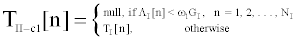(1)

where TII-c1[n] was the updated start time for the n-th cycle in Step II Criterion 1, “null” represented the action that the identification line was removed, ΛI[n] was the inspiratory excursion difference at the n-th cycle in Step I (see also Section III-B2), and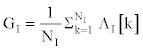was the ensemble average of inspiratory excursion calculated over all cycles identified in Step I. The threshold ω1 was chosen as 37% in the algorithm.

Criterion 2: The undesired cycles in Pattern 2 were located in the inspiratory phases. An example was the n-th cycle in Figure 9. It could be seen that the expiratory excursion (i.e. the difference between maximum and right minimum voltage) of the previous cycle (i.e. the (n-1)-th cycle) was much smaller compared to that of the (n-2)-th cycle. In this criterion, the ratio of expiratory excursions between the (n-2)-th and (n-1)-th cycles was compared with the ensemble average ratio. If the ratio was larger than the ensemble average, then the (n-1)-th cycle was identified as undesired and was removed. Similar to Criterion 1, after all the undesired cycles in this pattern were identified and removed, the cycles were renumbered according the updated results. Criterion 2 was expressed as: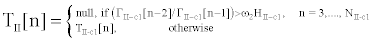(2)

Where ГII-c1[n] was the expiratory excursion difference at the n-th cycle in Step II-Criterion 1 (see also Section III-B2), and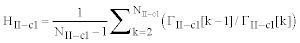was the average ratio of the expiratory excursions between a cycle and the previous one obtained in Criterion 1. The threshold ω2 was set as 8 in the algorithm. The start time for the n-th cycle obtained in Criterion 2 was the updated start time in Step II; therefore, notation TII[n] was used instead of TII-c2[n].

After implementing Criterion 2, all the undesired cycles were removed and the total number of desired cycles was determined. The updated start time for the n-th cycle was given by TII[n] in (2). However, as observed in Figure 9, the start times identified at Step II were not the exact start times of the cycles. The offsets usually represented very small amounts of time. A two-stage procedure was proposed in Step III to perform a final adjustment on the start times.

Step III: Adjustment of start time for the cycle: The offsets in start times specified in Step II often occurred if there were many small dips or ripples in the ending segments of expiratory phases. Consequently, the cycle identification line was drawn between these dips instead of at the correct desired start time of the cycle. As the ending segments in expiratory phases often were different, it was difficult to specify the exact start times by a single criterion. In general, start times were observed to have one or both of the following features: (a) the excursion voltage after the start time was consistently increasing, which could be regarded as an indication of the next inspiratory phase, and (b) the excursion voltage at the start time had a locally mathematical minimum or near-mathematical minimum (near-mathematical minimum meant a value that was so close to the mathematical minimum that the difference could not be detected visually). This motivated us to implement a two-stage procedure to perform a final adjustment on the cycle identification lines such that the exact start time for each cycle could be successfully determined.

In the first stage, the time after which the excursion voltage consistently increased was identified. Such times, if identified, happened to be the exact start times for most of the cycles. However, for the cycles in which the ending segment between the previous expiratory phase and next inspiratory phase had one or more dips, the start times obtained by the first stage were often over-adjusted. Stage 2 was introduced to undo the over-adjustment by identifying the dip with a local or visual minimum in the excursion of the expiratory phase. The two stages are described as follows.

Stage 1: In this stage, the time instants after which the excursion voltages increased consistently over a certain number of samples were identified. In the algorithm, 50 samples were chosen, which was half of a second (500 ms). If there existed some dips among those samples, the excursion voltages at those samples did not have a consistent increasing trend. As shown in the diagram for Stage 1 in Figure 10, a search was performed for each cycle using the start time obtained in Step II. If excursion voltages in the next 50 samples did not increase consistently, the cycle start time was increased by one sample period and another 50 samples were examined from the updated start time. This procedure was repeated until a time-instant was identified such that the excursion in the next 50 samples increased consistently.

The first plot in Figure 11 demonstrates the results from this stage. The start times for the n-th and (n+1)-th cycle were updated by vertical dotted lines to the times TIII-s1[n] and TIII-s1[n+1], respectively. It could be seen from the figure that start times for some cycles identified in Stage 1 were over-adjusted by a small time period from the correct desired start times. The reason was that there was often a dip in the end portion of the expiratory phase with an excursion voltage lower than the excursion at the start time specified in Stage 1. If the dip was deep enough (being a local or visual minimum), it was considered to be part of the inspiratory phase in the current cycle. Otherwise, it was considered to be part of the expiratory phase in the previous cycle.

In Stage 1, a start time was identified as a point at which excursion voltages in the next 50 samples increased consistently. As a result, all ripples or bumps were excluded from the inspiratory phases in current cycles and were included in the expiratory phases of previous cycles. Consequently, the start time was over-adjusted. Further adjustments were performed in Stage 2. Stage 2: In this stage, the over-adjusted start times for the cycles specified in Stage 1 were adjusted to the correct ones. As shown in the diagram of Stage 2 in Figure 10, the valley of a dip in the end portion of the expiratory phase was first located by finding the local minimum prior to the start time obtained in Stage 1. This was performed by a back-search for a decreasing trend in the excursion voltage until the time prior to which the voltage started increasing was found. Notation TIII-s2[n] was used to represent the time at which the valley was located. Next, if the valley in the dip was deep enough, the cycle identification line was moved to the valley. Using the local maximum voltage in the range from the valley to the start time obtained in Stage 1 as a reference, we checked if the voltage difference between the reference and that in the valley was much larger than the difference between the reference and that at the start time obtained in Stage 1. The ratio of the two voltage differences was used in the algorithm. If the ratio was larger than a threshold ω3 = 10, then the start time of the cycle was adjusted to the valley in the dip; otherwise, there was no adjustment. The local maximum was denoted by ФIII-s2[n] = max (Y(t), TIII-s2[n] ≤ t ≤ TIII-s2[n] ) . Stage 2 could be expressed by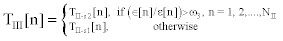(3)

where ε[n] = ФIII-s2[n]-Y (TIII-s2) was the voltage difference between the reference and that in the valley, and ε[n] = ФIII-s2 [n]-Y (TIII-s1) was the difference between the reference and the voltage at the start time obtained in Stage 1. The result from this stage is illustrated in the second plot in Figure 11. The final start times identified in Stage 2 in Step III were represented by solid lines at TIII[n] and TIII[n+1]. For comparison, the results from Stage 1 were also plotted as dotted lines at (III-s1[n+1]) and (TIII-s1[n]), respectively. It could be observed from the figure that the start times identified in Stage 2 indeed represented the correct desired start times.

Step IV: Distorted cycle detection: Even though the average shape of tidal breath cycles varied among and within participants, distorted cycles were characterized by one or more of the following when compared to typical tidal breath cycles: notably shorter durations, remarkably larger or smaller excursions, the presence of rapid signal deflections, and upward or downward trends within the signal that created a noticeable difference in the initial and final voltages. The distorted cycles could be detected by calculating the slope in each cycle, Ψ[n] = (Y (TIII[n+ 1])-Y (TIII[n] ))/(TIII[n+1]-TIII[n]). If the absolute value of a cycle’s slope was larger than a threshold ω4, which was set to 0.19 in the algorithm, the cycle was identified as distorted and was not used in further analysis. Figure 12 showed the results for the proposed scheme in distorted cycle detection. The distorted and non-distorted cycles were represented by dashed and solid signal traces, respectively. The slope in the n-th cycle was larger than ω4 and the slope in the (n + 1)-th cycle was smaller than -ω4. Both cycles were classified as distorted.

Figure 12: Step IV of Automatic Cycle Identification Algorithm. Distorted cycles were identified by the dashed line.

Simulation

We compared the results of the automatic cycle identification algorithm (ACIA) with those of the hand-coded method (HCM). The ACIA and HCM results were quantitatively compared using a sample of 811 tidal cycle start times across six different participants of the original twenty. The respiratory signals used for this comparison were a subset of those described in Section II but were not used in any way to develop or test the ACIA. The use of these signals allowed an unbiased assessment of the ACIA’s accuracy. To identify tidal breath cycles using hand coding, an experienced coder viewed each respiratory signal as a time waveform in the software program Time-Frequency Analysis for 32- bit Windows . Each cycle’s initial and final points were identified using the waveform information. The software’s label utility was used to mark the cycle’s boundaries. The precision of the measurement was increased by zooming in on the cycle’s initial and final points on separate passes and marking these points. The time required to code one signal by hand ranged approximately from 10 to 20 minutes, depending on the complexity of the signal. The automatic cycle identification algorithm (ACIA), described in Section III, was used to identify cycles in the same respiratory signals used for the handcoded method (HCM). The threshold values and constants applied in the algorithm are listed in table 1. The cycles identified by both ACIA (solid lines) and HCM (dashed lines) were plotted together on each respiratory signal (Figure 13). In some cases, both methods identified the same start time. In other cases, a zoomed-in review of the start times indicated that the ACIA more accurately identified the appropriate point than the HCM, due primarily to the resolution with which the algorithm could analyze the signal. In order to compare the ACIA with the HCM more exactly, we evaluated the duration of all cycles in the respiratory signals made by these two algorithms. A large-scale view of Figure 13 shows three examples in which the two methods identified the same cycle start times. When the signal resolution was increased, as in the inset in the upper left-hand corner of the figure, a discrepancy between the methods became apparent.

Parameter Meaning Value
ω1 Scalar of the substraction between maximum and left minimum excursion voltage of the n-th cycle. 0.37
ω2 Scalar of the substraction between maximum and right minimum excursion voltage of two adjacent cycles. 8
ω3 Threshold for modification of cycle identification lines in stage 2, Step III. 10
ω4 Threshold for distorted cycle detection. 0.19

Table 1: Criteria Parameters.

Figure 13: Example comparison of the Automatic Cycle Identification Algorithm and the Hand-Coded Method on a filtered signal. The inset shows a zoomed-in comparison.

To test the accuracy of the ACIA, two coders familiar with respiratory signals (the third author and a person not associated with the ACIA development) independently used the MATLAB software environment to view the ACIA and the HCM results side by side. The coders examined which method more accurately identified the cycle start times, given the placement of the start times to the physiological signals. In all 811 cases, the AICA either more accurately identified the correct start time of the cycle than did the HCM, or replicated the HCM’s results. The HCM was unable to resolve inspiratory start times as well as the ACIA.

To quantify the difference between the results obtained by the ACIA over HCM, the coders also calculated the time difference (ms) between the two methods for each of the 811 cycles. Figure 14 shows the time differences across the cycles. It was found that the ACIA results were identical to those of the HCM results in 68 cycles among the total cycles, which is 68/811 = 8.38%. ACIA results were 10 to 50 ms more accurate than those of the HCM in 622 cycles (76.70%), 60 to 100 ms more accurate in 72 cycles (8.88%), 110 to 360 ms more accurate in 41 cycles (5.06%), and 370 to 800 ms more accurate in 8 cycles (0.99%). Figure 15 shows the distribution of the time differences and frequencies at which the ACIA was more accurate than the HCM.

Figure 14: Difference between the Automatic Cycle Identification Algorithm and the Hand-Coded Method (811 cycles).

Figure 15: Distribution of differences and frequencies at which the Automatic Cycle Identification Algorithm is more accurate than Hand-Coded Method.

#### Conclusion

We developed a novel technique for automatically identifying respiratory cycles. Given the complexity of the respiratory signals, the results of the automatic cycle identification algorithm (ACIA) are promising. The algorithm surpassed the hand-coded method. It provided not only a time-saving method for coding respiratory signals, but also improved upon the accuracy with which the individual cycles were identified. In summary, compared to the results performed by an experienced hand-coder, the ACIA was able to more quickly select tidal breath cycles, more accurately identify and code the cycles’ start times so that they could be used in subsequent analyses.

#### References

Select your language of interest to view the total content in your interested language

### Article Usage

• Total views: 13082
• [From(publication date):
February-2012 - Sep 23, 2019]
• Breakdown by view type
• HTML page views : 9280

## Post your commentCan't read the image? click here to refresh
###### Peer Reviewed Journals

Make the best use of Scientific Research and information from our 700 + peer reviewed, Open Access Journals

International Conferences 2019-20

Meet Inspiring Speakers and Experts at our 3000+ Global Annual Meetings

Top